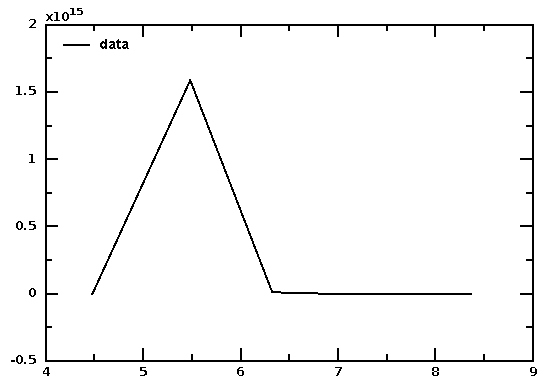# DMelt:Numeric/8 Numeric Python

Limitted access. First login to DataMelt if you are a full DataMelt member. Then login to HandWiki as a user.

# Numeric Python

JNumeric is a collection of extension modules to provide high-performance multidimensional numeric arrays to the Python programming language. This package closely follows NumPy CPython package which is described in NumPy Tutorial. There is a however a number of differences discussed below. Since JNumeric is implemented in Java, you can access Java libraries.

Here is a simple example how to with arrays. Let us find all packages of JNumeric:

import jnumeric
dir(jnumeric)

As example, consider "JNumeric" that provides high-performance arrays:

import jnumeric.JNumeric as np
a = np.array(10)
b = np.array( [[1,0],[0,1]],'f') # assume float
print b

The output is

[[1.0 0.0]
[0.0 1.0]]


Note that types of arrays can be defined as usual. The following types are supported: Float, Float32, Float64, Int, Int16, Int32, Int64, Int8

import jnumeric.JNumeric as np
a = np.ones(3, np.Int32)

You can do the usual math with vectors and matrices:

import jnumeric.JNumeric as np
b=np.array( [[1,3],[2,3]],'f')
c=np.array( [[2,1],[4,10]],'f')
d1=b*c; d2=b+c; d3=b/c # multiply, add, divide
print d1, d2,d3
 [[2.0 3.0]
[8.0 30.0]] [[3.0 4.0]
[6.0 13.0]] [[0.5 3.0]
[0.5 0.300000011921]]


The usual NumPy methods are also available:

import jnumeric.JNumeric as np
b=np.array( [[1,3],[2,3]],'f')
print b.itemsize()
print zeros( (3,4) )
print ones( (2,3,4), 'f')

Print methods associated with array a as:

print dir(a)

DataMelt allows to interface JNumeric fast arrays with other Java classes and graphical canvases for plotting similar to CPython when interfacing NumPy with MathPlot. For example, this script generate two arrays, transform them to sqrt() (for X) and exp() (for Y) and plot on interactive canvas: We use the Java classesjhplot.P1D andjhplot.HPlot to perform this task:

The output image isThe package also provides FFT ( fast Fourier transform) and LinAlg (Linear algebra) modules:

and the output is "[(2+0j) 0j]". It also provides an extension of the math module:

>>> from  jnumeric import Umath
>>> print dir(Umath)
[ 'add', 'arccos', 'arccosh', 'arcsin', 'arcsinh', 'arctan',
'arctanh', 'bitwise_and', 'bitwise_or', 'bitwise_xor', 'ceil',
'classDictInit', 'conjugate', 'cos', 'cosh', 'divide', 'equal',
'exp', 'floor', 'greater', 'greater_equal', 'imaginary', 'less',
'less_equal', 'log', 'log10', 'logical_and', 'logical_not', 'logical_or',
'logical_xor', 'maximum', 'minimum', 'multiply', 'not_equal', 'power',
'real', 'remainder', 'sin', 'sinh', 'sqrt', 'subtract', 'tan', 'tanh']

Note that "jnumeric" package cannot be used inside pure Java programs since it calls Jython classes. Here is a more complex scripts that tests matrix multiplications, transformations and some linear algebra operations:

# Differences between JNumeric and NumPy

• There is no multiarray module.
• Uumath is a submodule of the Numeric package.
• Ones acts like zeros if no typecode is given instead of defaulting to Int.
• Int refers to native (Int32) int type rather than largest (Int64) type.
• a doesn't work for zeros sized arrays -- use a[()] or a[...].
• Some of the unfuncs that should have been returning ints, returned types that matched their arguments (logical_and for example). Also, some of the documented ufuncs are missing from CNumeric.
• Changed the behavior of dot. Now dot acts on axis -1/0 by default, since it now accepts two axes arguments, this is not as big a deal as it once was.
• Astype does not make a copy if the type matches the current type. (This matches the behavior of asarray).
• There are several functions missing that should be added: cross_correlate, sarray, dump, dumps, load, loads (pickle functions) divide_safe, negative, invert, left_shift, right_shift, fmod, hypot, around, sign